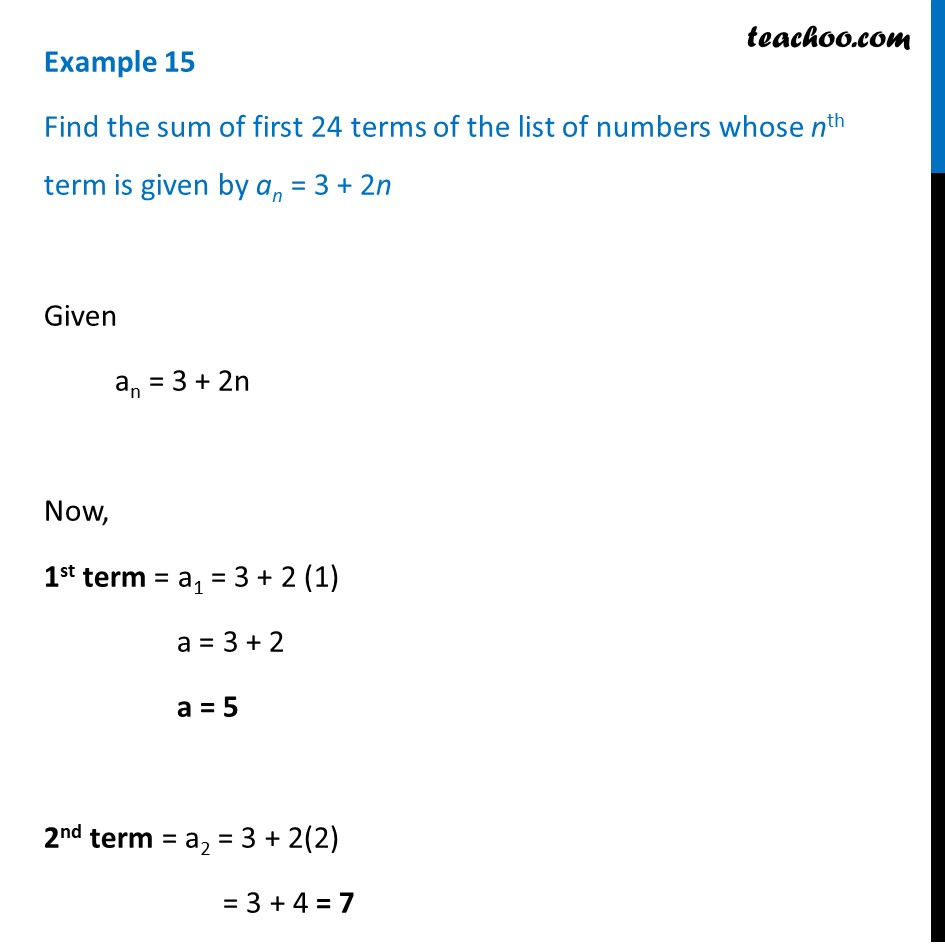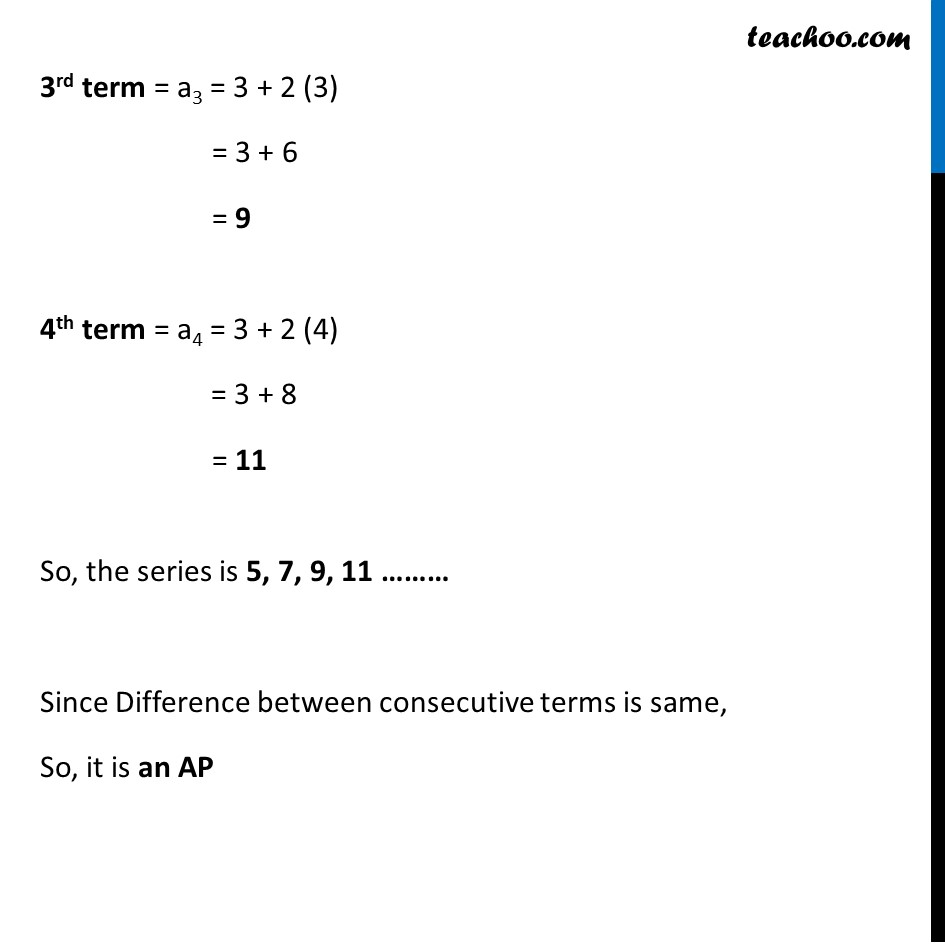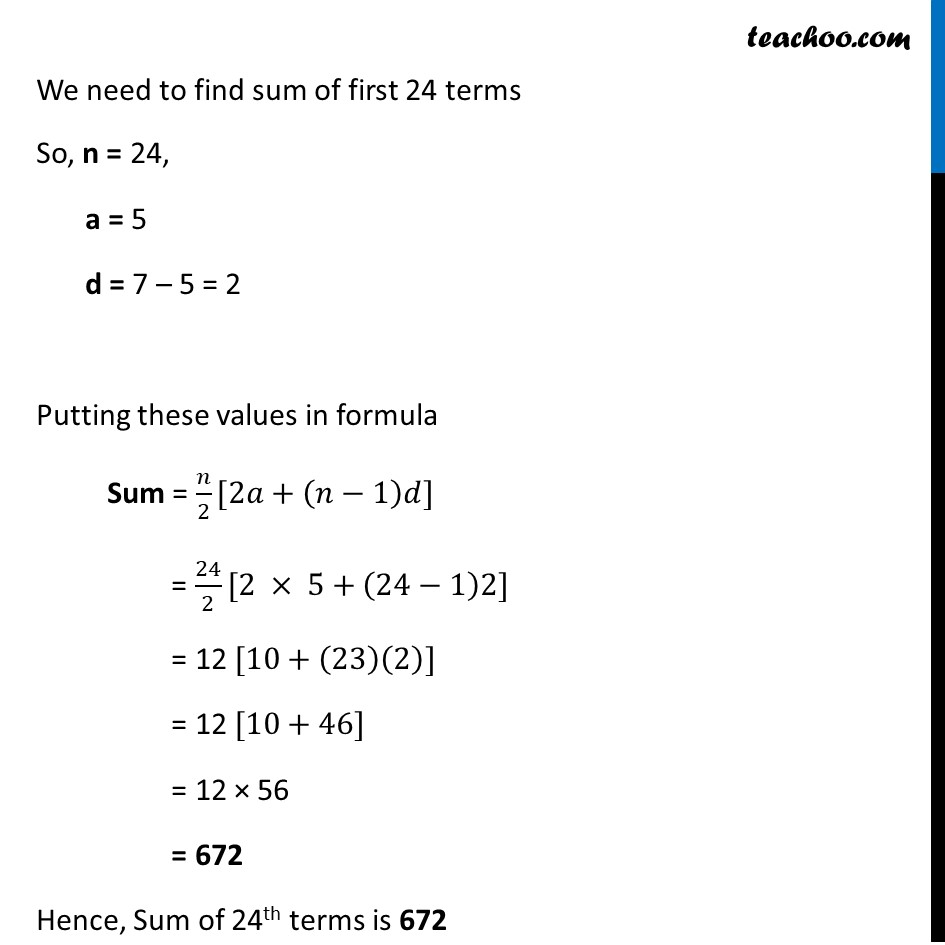Examples

Chapter 5 Class 10 Arithmetic Progressions
Serial order wiseLearn in your speed, with individual attention - Teachoo Maths 1-on-1 Class

### Transcript

Example 15 Find the sum of first 24 terms of the list of numbers whose nth term is given by an = 3 + 2n Given an = 3 + 2n Now, 1st term = a1 = 3 + 2 (1) a = 3 + 2 a = 5 2nd term = a2 = 3 + 2(2) = 3 + 4 = 7 3rd term = a3 = 3 + 2 (3) = 3 + 6 = 9 4th term = a4 = 3 + 2 (4) = 3 + 8 = 11 So, the series is 5, 7, 9, 11 ……… Since Difference between consecutive terms is same, So, it is an AP We need to find sum of first 24 terms So, n = 24, a = 5 d = 7 – 5 = 2 Putting these values in formula Sum = 𝑛/2[2𝑎+(𝑛−1)𝑑] = 24/2[2 × 5+(24−1)2] = 12 [10+(23)(2)] = 12 [10+46] = 12 × 56 = 672 Hence, Sum of 24th terms is 672